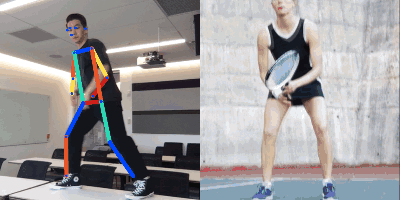## 1. PoseNet

Google Creative Lab 所制作Move Mirror 应用的核心是姿态估计（pose estimation）模型.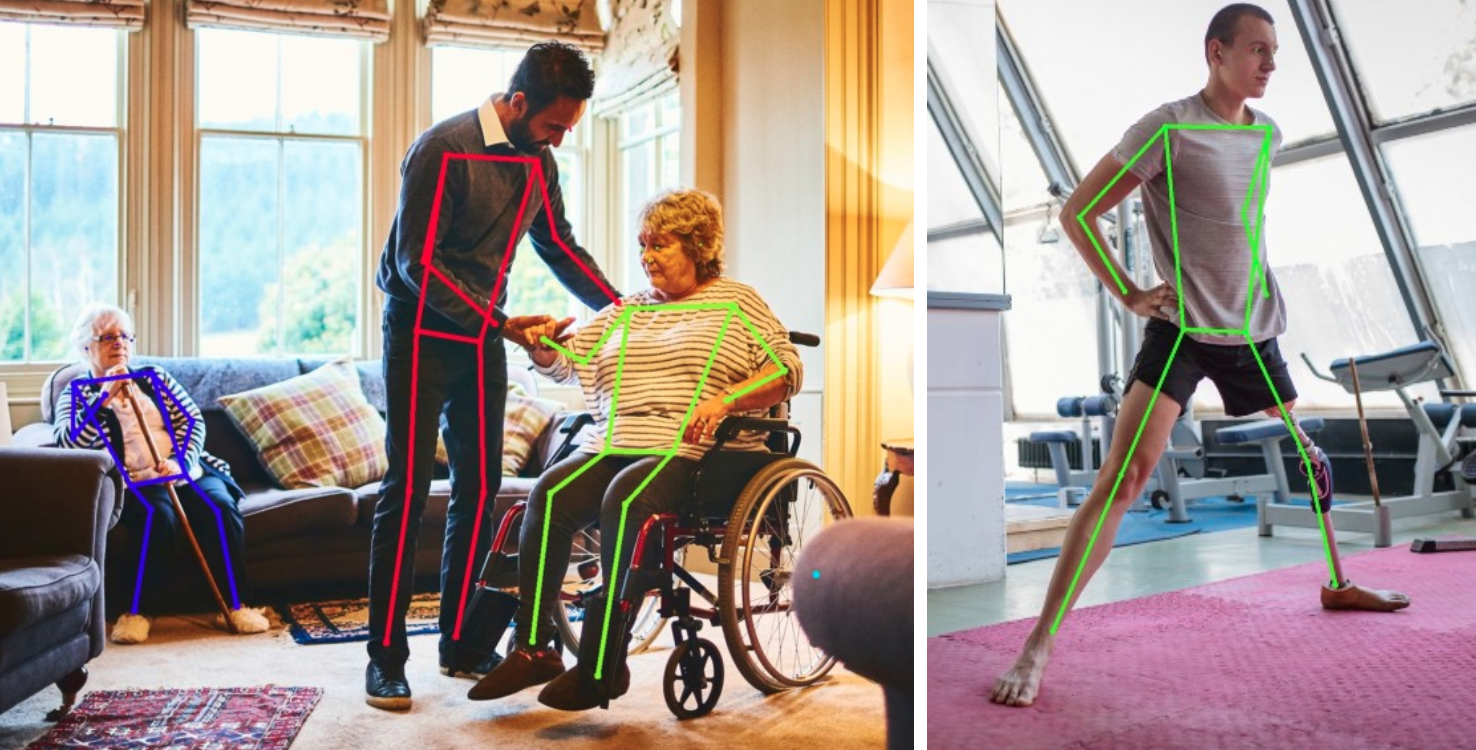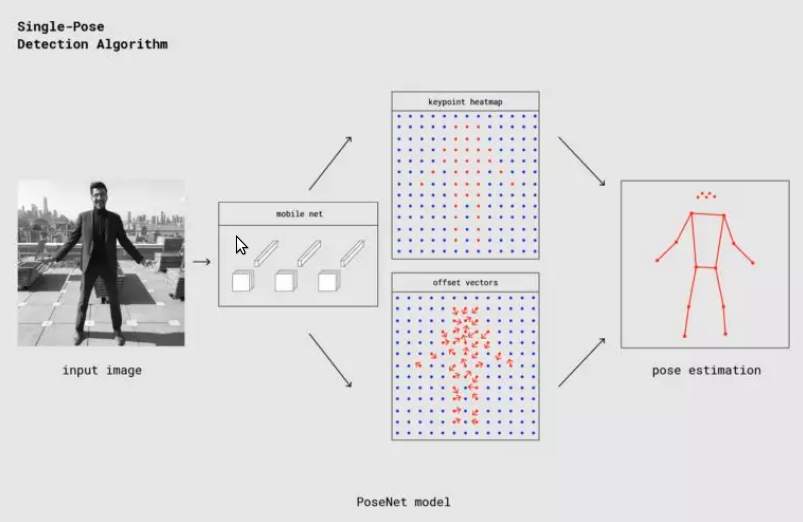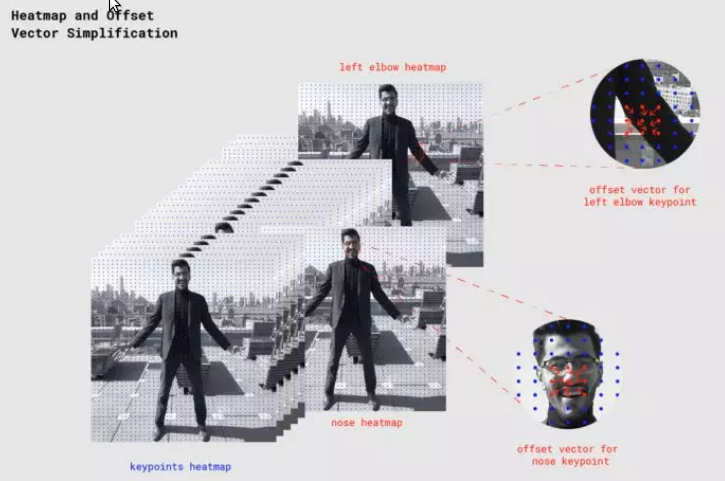Move Mirror 灵感源：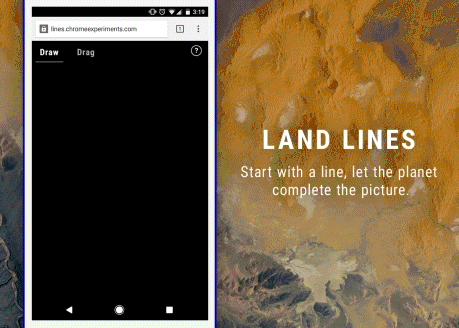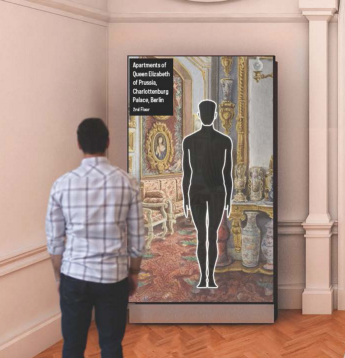## 2. MoveMirror 构建

Move Mirror 团队选择 PoseNet作为应用背后的模型. 在原型开发阶段，团队通过简单的web API访问PoseNet模型，这极大地简化了原型开发流程. 只需向内部的 PoseNet API 接口发送一个HTTP POST请求，提交base64编码的图像，API就会传回姿态数据（基本无延迟）. 若干行JavaScript代码，一份API密钥，搞定！

### 2.1. 构建数据集

 - 多样性 为了更好地匹配用户做出的各种各样的动作，图像的姿态需要尽可能多样化.

 - 全身像 从用户体验的一致性出发，决定只使用全身像.### 2.2. 姿态匹配

PoseNet的姿态数据包括17个关键点的坐标，以及相应的置信度.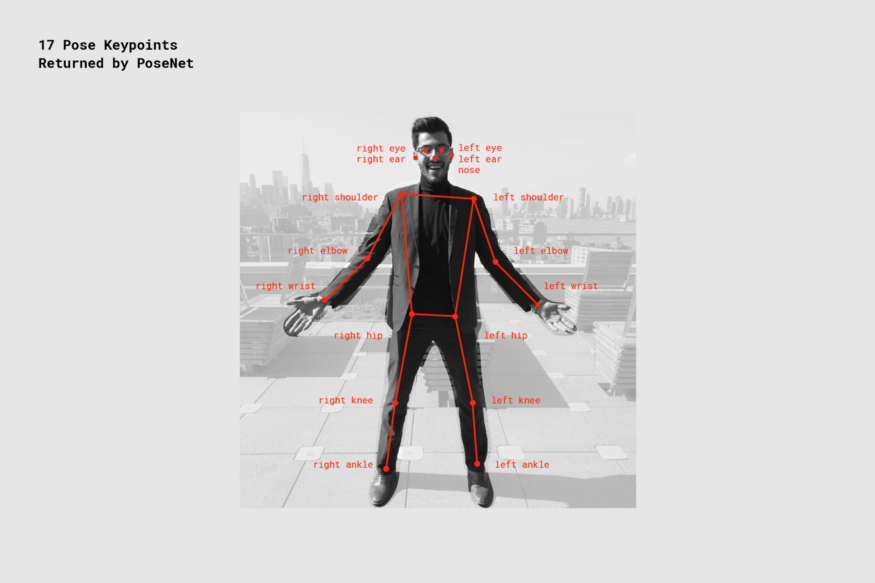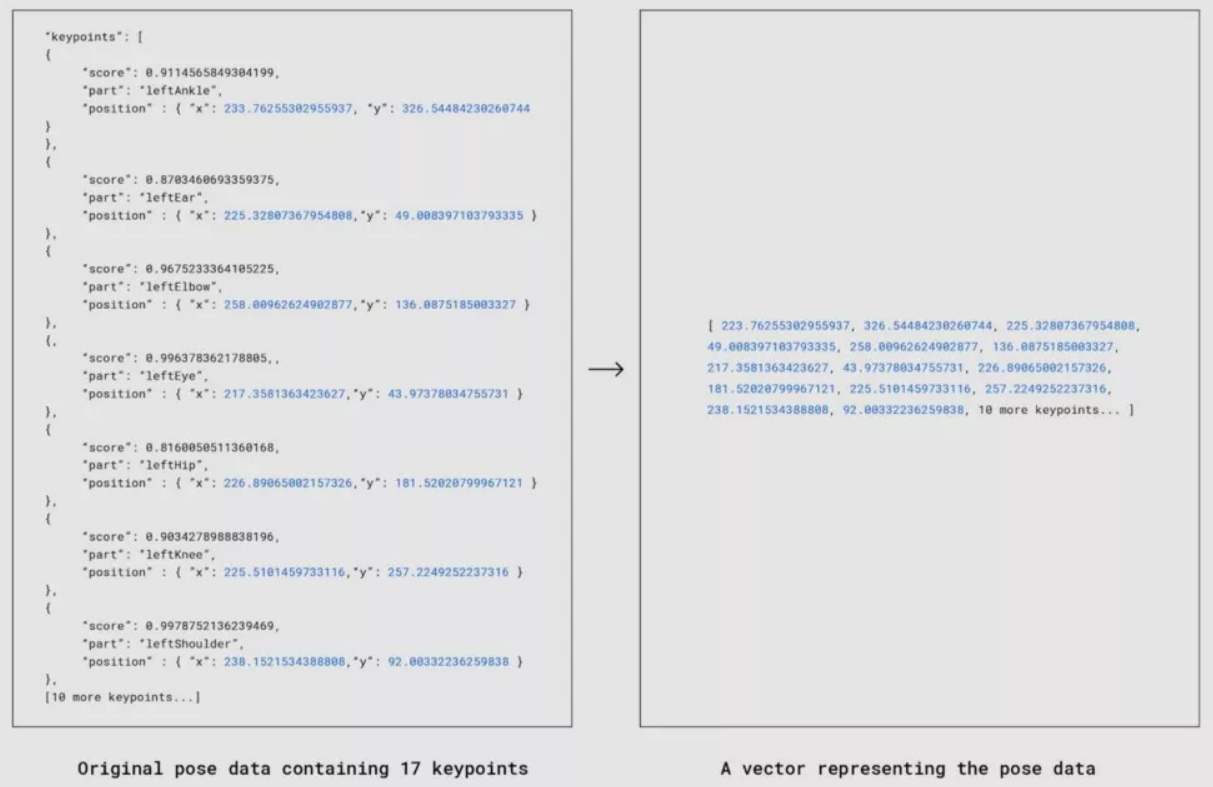#### 2.2.1. 余弦相似度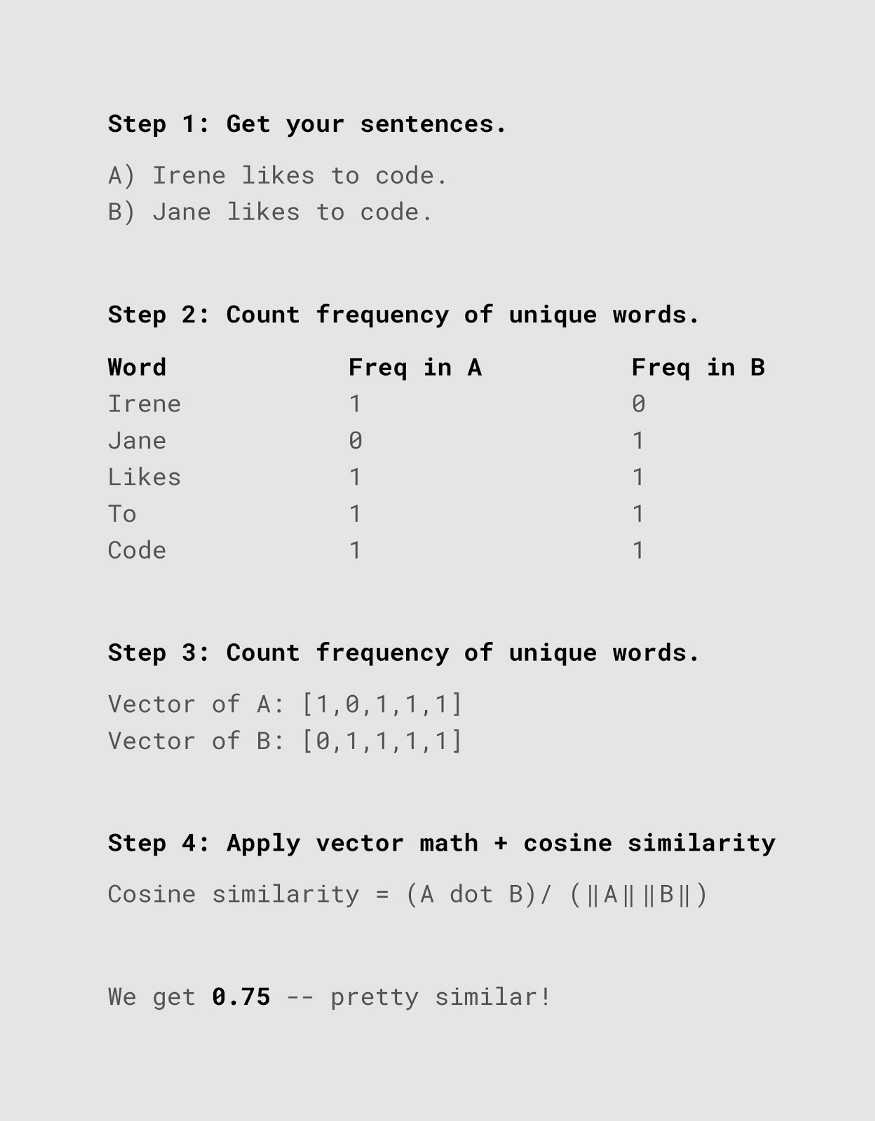- 缩放 - 根据每个人体的边界框坐标进行裁剪，并将每张图片缩放到固定尺寸(对应的关键点坐标也同样处理)；

 - 归一化 - 通过将缩放后的关键点坐标作为 L2 正则化向量(各分量的平方和等于1)来进行归一化.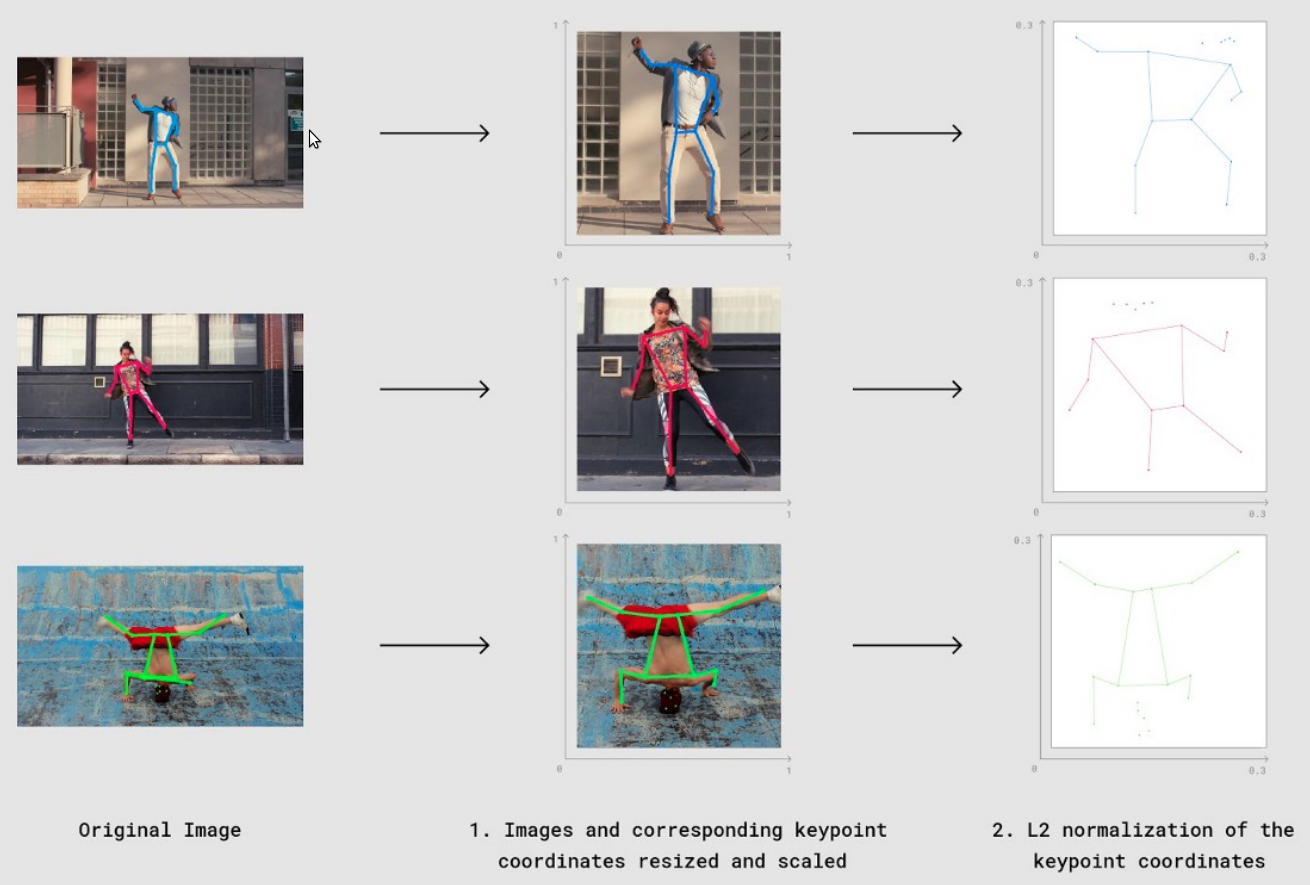$$D(F_{xy}, G_{xy}) = \sqrt{2 * (1 - cosineSimilarity(F_{xy}, G_{xy}))}$$

javascript 计算如 - moveMirrorCosineDistance.js

// Great npm package for computing cosine similarity
const similarity = require('compute-cosine-similarity');

// Cosine similarity as a distance function. The lower the number, the closer // the match
// poseVector1 and poseVector2 are a L2 normalized 34-float vectors (17 keypoints each
// with an x and y. 17 * 2 = 34)
function cosineDistanceMatching(poseVector1, poseVector2) {
let cosineSimilarity = similarity(poseVector1, poseVector2);
let distance = 2 * (1 - cosineSimilarity);
return Math.sqrt(distance);
}

#### 2.2.2. 置信度加权匹配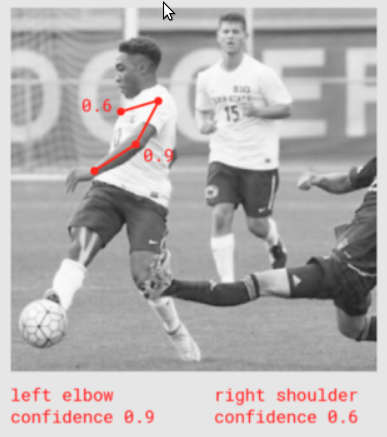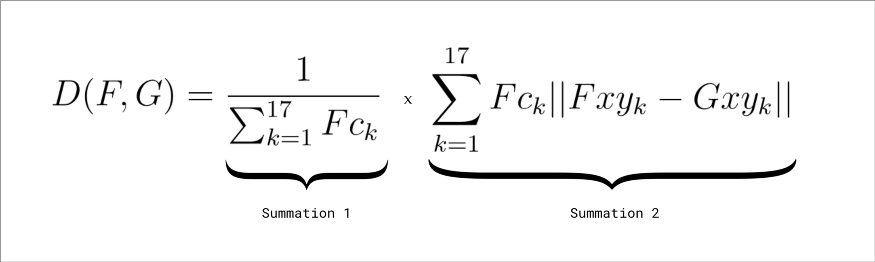javascript 计算如 - moveMirrorWeightedDistance.js

// poseVector1 and poseVector2 are 52-float vectors composed of:
// Values 0-33: are x,y coordinates for 17 body parts in alphabetical order
// Values 34-51: are confidence values for each of the 17 body parts in alphabetical order
// Value 51: A sum of all the confidence values
// Again the lower the number, the closer the distance

function weightedDistanceMatching(poseVector1, poseVector2) {
let vector1PoseXY = poseVector1.slice(0, 34);
let vector1Confidences = poseVector1.slice(34, 51);
let vector1ConfidenceSum = poseVector1.slice(51, 52);

let vector2PoseXY = poseVector2.slice(0, 34);

// First summation
let summation1 = 1 / vector1ConfidenceSum;

// Second summation
let summation2 = 0;
for (let i = 0; i < vector1PoseXY.length; i++) {
let tempConf = Math.floor(i / 2);
let tempSum = vector1Confidences[tempConf] * Math.abs(vector1PoseXY[i] - vector2PoseXY[i]);
summation2 = summation2 + tempSum;
}

return summation1 * summation2;
}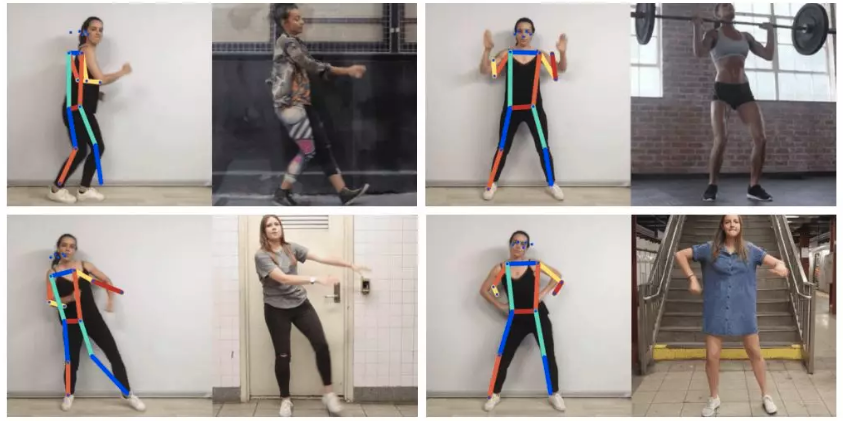### 2.3. 匹配速度优化

Move Mirror 选用的数据结构是 VP树(vantage-point tree).

#### 2.3.1. VP-Tree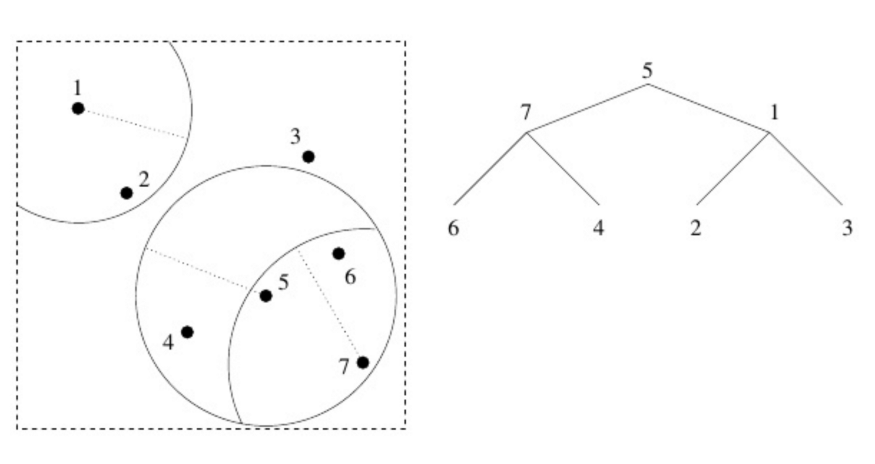- 在数据点中选取一点（可以随机选取）作为根节点（上图中为5）；

 - 绕着5画一个圈，将空间分割成圈内和圈外两部分;

 - 接着, 在圈内、圈外各选一点作为制高点（上图中为71）；

 - 然后，绕着每个制高点各画一个圈，同样在圈内、圈外各选一点……

VPTree 实现 - moveMirrorVPTree.js

const similarity = require('compute-cosine-similarity');
const VPTreeFactory = require('vptree');

const poseData = [ […], […], […], …] // an array with all the images’ pose data
let vptree ; // where we’ll store a reference to our vptree

// Function from the previous section covering cosine distance
function cosineDistanceMatching(poseVector1, poseVector2) {
let cosineSimilarity = similarity(poseVector1, poseVector2);
let distance = 2 * (1 - cosineSimilarity);
return Math.sqrt(distance);
}

function buildVPTree() {
// Initialize our vptree with our images’ pose data and a distance function
vptree = VPTreeFactory.build(poseData, cosineDistanceMatching);
}

findMostSimilarMatch(userPose) {
// search the vp tree for the image pose that is nearest (in cosine distance) to userPose
let nearestImage = vptree.search(userPose);

console.log(nearestImage.d) // cosine distance value of the nearest match

// return index (in relation to poseData) of nearest match.
return nearestImage.i;
}

// Build the tree once
buildVPTree();

// Then for each input user pose
let currentUserPose = [...] // an L2 normalized vector representing a user pose. 34-float array (17 keypoints x 2).
let closestMatchIndex = findMostSimilarMatch(currentUserPose);
let closestMatch = poseData[closestMatchIndex];

Move Mirror仅仅返回最匹配用户姿态的图像. 不过，通过遍历VP-Tree，不难返回更多结果，比如最接近的10张或20张图像. 这可以用来制作调试工具，发现数据集的问题.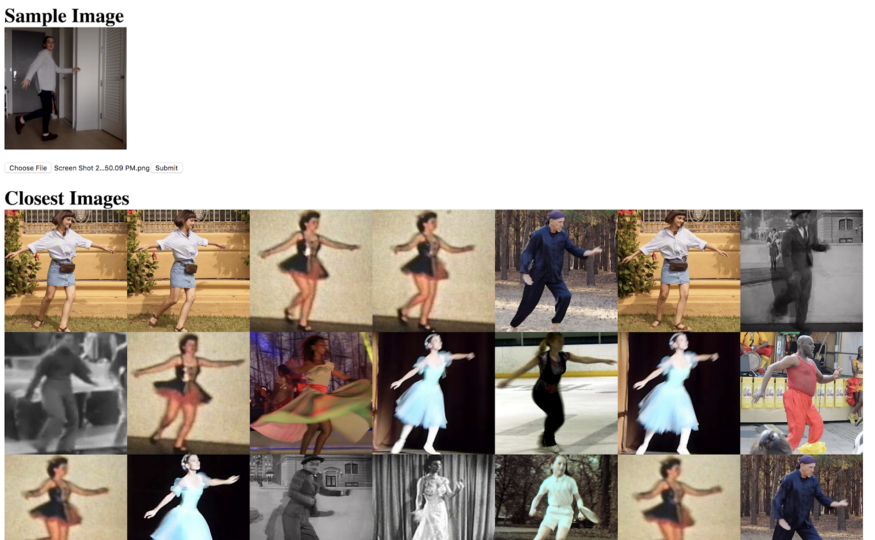## 3. 结语

Move Mirror团队期待能看到更多类似的有趣应用，比如匹配舞蹈动作，匹配经典电影片段. 或者反向操作，基于姿态估计帮助人们在家中练习瑜伽或者进行理疗.

Last modification：June 9th, 2020 at 11:18 am